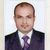# QlikView App Dev

Discussion Board for collaboration related to QlikView App Development.

Announcements
cancel
Showing results for
Did you mean:Creator II

## AVG calculation

Dear All,

I am calculating AVG

=sum(Dollars)/Count(standardID) (60663/2823)=21%

I have a doubt is this correct way to calculate AVG

Niranjan

1 Solution

Accepted SolutionsMVP

Second one is seems right but without see the whole data not confirm you need to check from your side. Also as Henric suggest do not use Aggr and use PersonId in place of Standard ID but if the Personid has same Id as the Standard Id then use that.

12 RepliesCreator

Try this

Avg(Aggr(sum(Dollars)/Count(standardID), Dimension))

AleMVP

Updated the details now

Sorry i forget to add the dimension details

Check your output with the expected manual result if it gives result then it will correct other wise you can use Avg function for this. And if the fields which is in Numerator or denominator has proper relation then it will correct for you.

You can also try with

=Aggr( sum(Dollars)/Count(standardID), DimensionName )

Or

=Avg( Aggr( sum(Dollars)/Count(standardID), DimensionName ))Partner

Hi,

21% is wrong. What your result means is an average of  21.49 Dollars per standardID.

EduardoCreator II
Author

Dear All,

AVG Occupancy cost per Person

=sum(Dollars)/Count(standardID)

=60663/2823

How can i calculate AVG of cost per person

Best regards,

NiranjanCreator

Avg(Aggr(sum(Dollars)/Count(standardID), Person))

RegardsEmployee

It depends what you want to calculate. Dollars per standardID? Then Sum(Dollars)/Count(distinct standardID). Note the "distinct".

Or per something else? See more on Average – Which average?

HICCreator II
Author

AVG Occupancy cost per person i want to calculate

i have only two dimension

how can i calculate this secnario

BEst Regards,

NiruMVP

If you calculate this in any chart then use

Dimension:- Person

Exper :- =Avg( (sum(Dollars)/Count(standardID) ) )

And if calculate this in any text box then

=Avg(Aggr(sum(Dollars)/Count(standardID), Person))Employee

Then you should calculate total cost and divide by number of persons. Hence:

Sum(Dollars) / Count( distinct PersonID )

Aggr is not needed here.

HIC